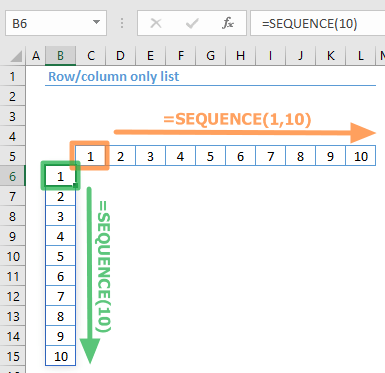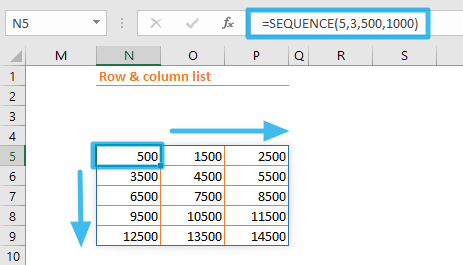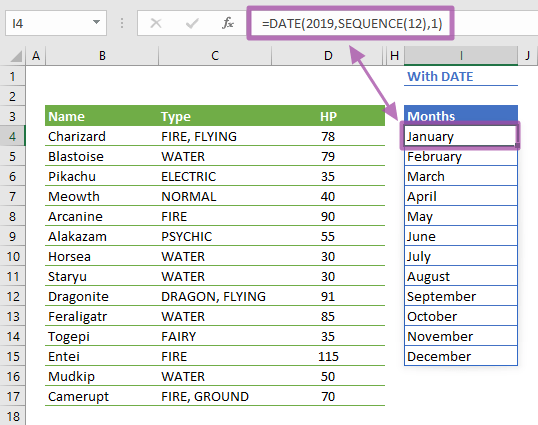The SEQUENCE function is a Math & Trigonometry formula that generates a list of sequential numbers in the form of an array or range. In this guide, we’re going to show you how to use the SEQUENCE function and also go over some tips and error handling methods.

The Dynamic Array functions can populate an array of values in a range of cells based on a formula. This behavior is called spilling and can help overcome the limitations of array formulas.

# Supported versions

• At the time of writing this article, Microsoft announced that this formula is currently only available to a number of select insider users. When it's ready, the feature is planned for release for Office 365 users.

# Syntax

=SEQUENCE(rows,[columns],[start],[step])

# Arguments

 rows The number of rows to be returned. [columns] Optional. The number of columns to be returned. The default value is 1. [start] Optional. Starting value. The default value is 1. [step] Optional. The increment of each step between values. The default value is 1.

Examples

## Row/column only list

Populate only rows: =SEQUENCE(10)

Populate only columns: =SEQUENCE(1,10)

formulas demonstrate a basic use case of the SEQUENCE function. Entering only a row argument populates only rows with incremental numbers starting from 1. The increment amount is 1 here, because the [step] argument was omitted.

Set rows to 1 and enter a number for the [columns] argument to populate only columns.## Row & column list

=SEQUENCE(5,3,500,1000)
formula demonstrates using both the rows and [columns] arguments to generate a two-dimensional range. The [start] and the [step] arguments determine where the sequence starts (from 500) and increment amount (from 1000) respectively.## Combination with other functions

=INDEX(Pokemon,SEQUENCE(5),SEQUENCE(1,2))
formula shows how to use dynamic array formulas and the SEQUENCE function in combination. To create a table with the INDEX function, you would need a helper column and rows that have the same index numbers before the SEQUENCE function. With this approach, all you need to do is generate arrays for row and column indexes with the SEQUENCE function. With the help of the SEQUENCE function, the INDEX function will act like a dynamic array function and populate the entire range.# Tips

• Using a dynamic array function like SEQUENCE with another function makes the formula return a spill. For example, you can use the following formula to generate a list of months.
=DATE(2019,SEQUENCE(12),1)• The SORT function shows an example that lists the top 3 results from a table.

# Issues

## #SPILL!

If there isn't enough space for adding the results below the formula, you will see a #SPILL! error. Excel marks the target range with dashed lines. Clear the contents of the cells in this range, and Excel will automatically update the results.## #VALUE!

If any of the arguments is not a numeric value, you will get a #VALUE! error.